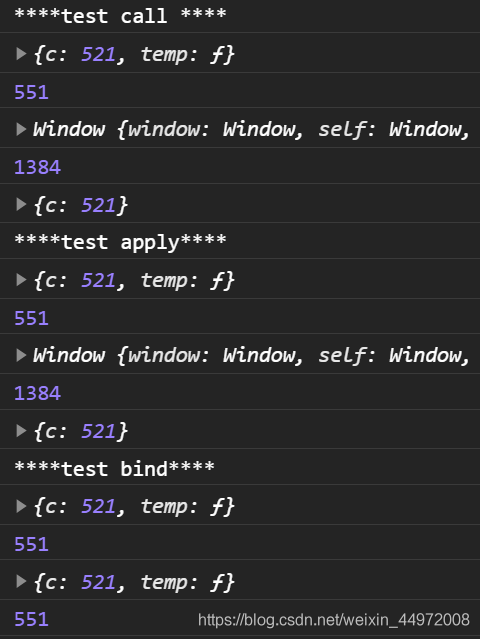# 【JS】JavaScript基礎知識自我檢查大過關（第三關）函式定義與呼叫

theme: fancy highlight: a11y-dark

「這是我參與11月更文挑戰的第28天，活動詳情檢視：2021最後一次更文挑戰

# 1. 函式定義的幾種方式

## 1.1 函式宣告式

```javascript function fn(a, b) { return a + b; }```

## 1.2 函式表示式

```javascript let fun = function(a, b){ return a + b; }```

## 1.3 建構函式式

```javascript let fun = new Function('a', 'b', 'return a + b')```

## 1.4 箭頭函式

```javascript let fn = (a, b) => { return a + b; };``` 關於箭頭函式的更多內容，請見【ES6】JavaScript函式-箭頭函式-this指向-簡寫

# 2. 函式呼叫的幾種方式

## 2.1 普通函式

```javascript fun() fun.call()```

## 2.2 物件的方法

```javascript obj.fun()```

## 2.3 建構函式

```javascript new Fun()```

# 4. 改變函式的this指向

## 4.1 使用call方法

```javascript fun.call(thisArg, arg1, arg2, ...)```

```javascript function add(a, b) { console.log(a + b) }

let obj = { c: 520 } add(1,2) // this指向window add.call(obj, 1, 2) // this指向obj ```

### 應用

```javascript function Father(uname, age, sex) { this.uname = uname this.age = age this.sex = sex }

function Son(uname, age, sex){ Father.call(this, uname, age, sex) }

let son = new Son('YK菌', 18, '男') console.log(son) // Son {uname: "YK菌", age: 18, sex: "男"} ```

## 4.2 使用apply方法

```javascript fun.apply(thisArg, [argsArray])```

let obj = { c: 520 } add(1,2) // this指向window add.call(obj, [1, 2]) // this指向obj ```

### 應用

```javascript let arr = [1, 34, 556, 44, 23] Math.max.apply(null, arr); // null 表示不需要改變this指向 Math.max.apply(Math, arr); // 不改變的話最好就指回去```

## 4.3 使用bind方法

```javascript fun.bind(thisArg, arg1, arg2, ...)```

```javascript function add(a, b) { console.log(a + b) }

let obj = { c: 520 } add(1,2) // this指向window let f = add.bind(obj, 1, 2) // this指向obj， 不會呼叫 f() // 呼叫函式 this指向obj ```

### 應用

```javascript let btn = document.querySelector('button') btn.onclick = function() { this.disabled = true setTimeout(function(){ this.disabled = false; }.bind(this), 3000) }```

## 4.4 區別與聯絡

### 區別

`call``apply` 會呼叫函式, 並且改變函式內部this指向. `call``apply` 傳遞的引數不一樣, `call` 傳遞引數 `arg1, arg2...`形式 `apply` 必須陣列形式`[args]` `bind` 不會呼叫函式, 可以改變函式內部`this`指向.

### 主要應用場景

`call` 經常做繼承. `apply` 經常跟陣列有關係. 比如藉助於數學物件實現陣列最大值最小值 `bind` 不呼叫函式,但是還想改變this指向. 比如改變定時器內部的`this`指向

# 5. 自定義call、apply、bind方法

## call.js

```javascript export default function call(Fn, obj, ...args) { if (obj === undefined || obj === null) { // 表示全域性物件（ES11新增特性） obj = globalThis; } // 為 obj 新增臨時的方法 obj.temp = Fn; // 呼叫 temp 方法 let result = obj.temp(...args); // 刪除tempfangfa delete obj.temp; // 返回執行結果 return result; }```

## apply.js

```javascript export default function apply(Fn, obj, arr) { if (obj === undefined || obj === null) { obj = globalThis; } // 為obj新增臨時方法 obj.temp = Fn; // 執行方法 let result = obj.temp(...arr); // 刪除臨時屬性 delete obj.temp; // 返回結果 return result; }

```

## bind.js

```javascript import call from './call.js'

export default function bind(Fn, obj, ...args) { // 返回一個新的函式 return function (...args2) { // 執行 call 函式、 return call(Fn, obj, ...args, ...args2); }; }

```

## 測試

```javascript import call from "./function/call"; import apply from "./function/apply"; import bind from "./function/bind";

console.log("*test call *");

function add(a, b) { console.log(this); return a + b + this.c; } let obj = { c: 521, }; window.c = 1314;

console.log(call(add, obj, 10, 20)) console.log(call(add, null, 30, 40))

console.log(obj) console.log("*test apply*"); console.log(apply(add, obj, [10, 20])) console.log(apply(add, null, [30, 40]))

console.log(obj) console.log("*test bind*"); let fn = bind(add, obj, 10, 20); console.log(fn());

let fn2 = bind(add, obj); console.log(fn2(10, 20)); ```